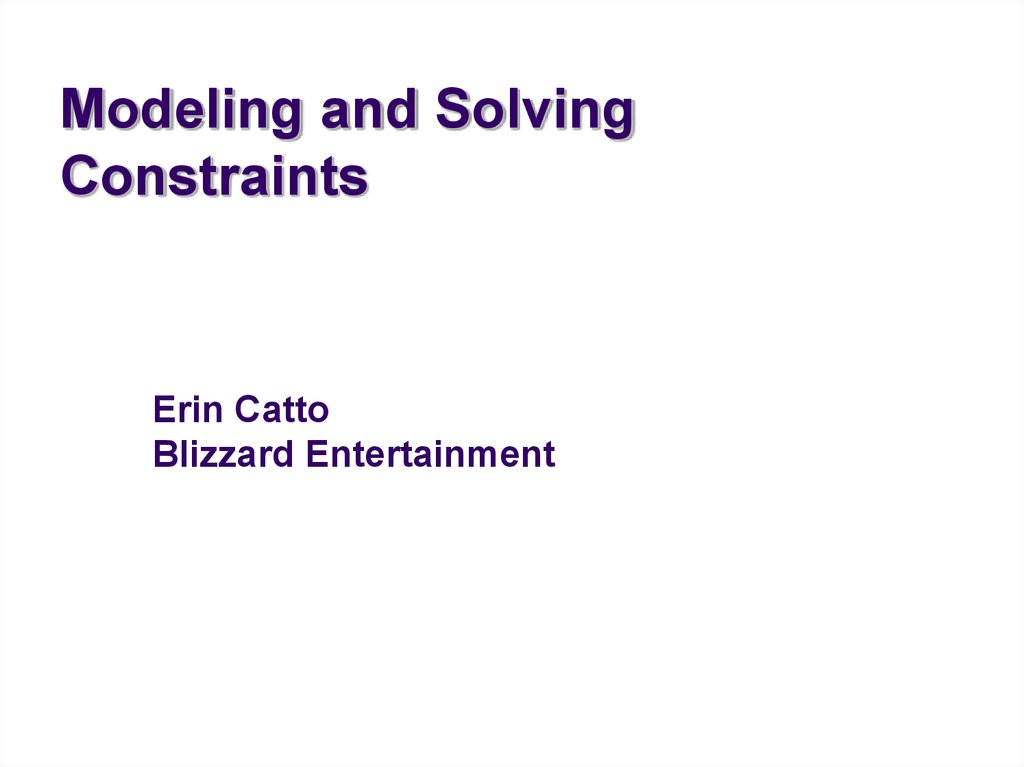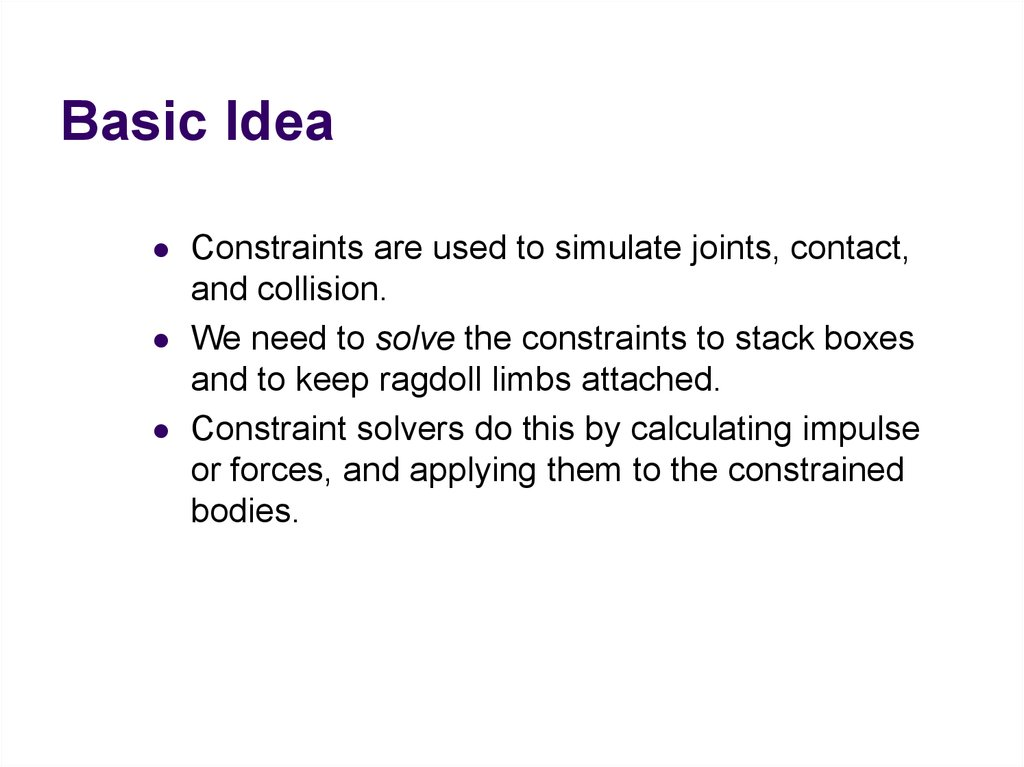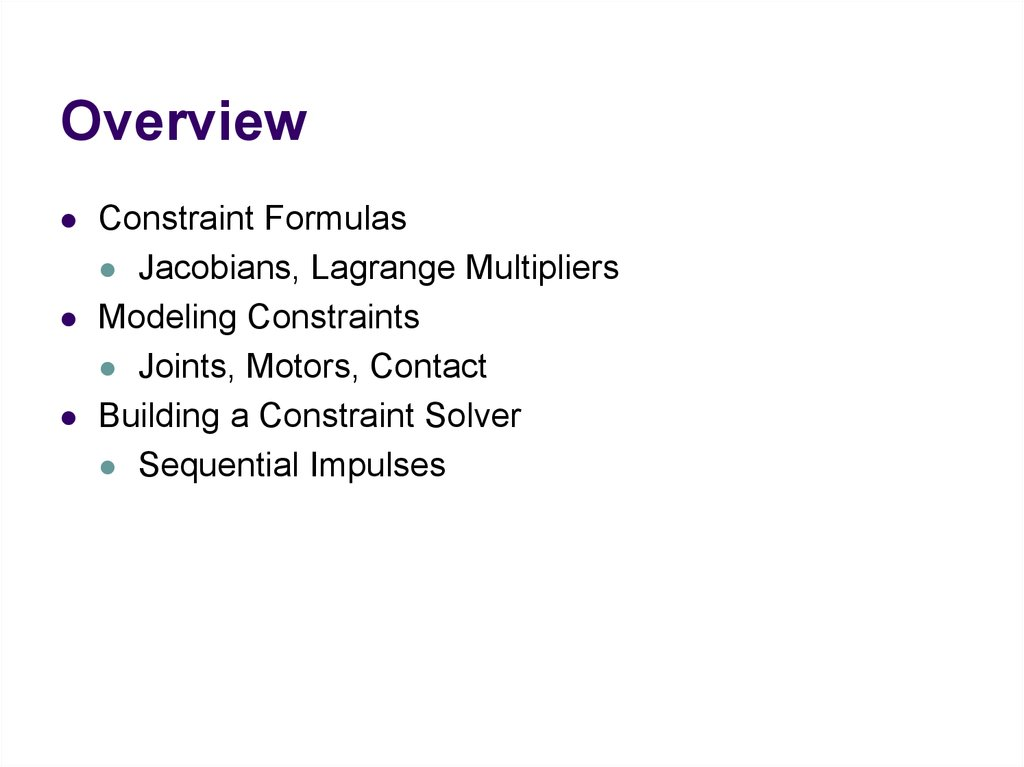# Modeling and Solving Constraints. Basic Idea

## 1. Modeling and Solving Constraints

Erin Catto
Blizzard Entertainment

## 2. Basic Idea

Constraints are used to simulate joints, contact,
and collision.
We need to solve the constraints to stack boxes
and to keep ragdoll limbs attached.
Constraint solvers do this by calculating impulse
or forces, and applying them to the constrained
bodies.

## 3. Overview

Constraint Formulas
Jacobians, Lagrange Multipliers
Modeling Constraints
Joints, Motors, Contact
Building a Constraint Solver
Sequential Impulses

## 4. Constraint Types

Contact and Friction

Ragdolls

## 6. Constraint Types

Particles and Cloth

## 8. Bead on a 2D Rigid Wire

Implicit Curve Equation:
This is the position constraint.
C ( x, y ) 0

## 9. How does it move?

The normal vector is perpendicular to the velocity.
n
v
dot(n, v) 0

## 10. Enter The Calculus

Position Constraint:
C ( x) 0
x
x
y
If C is zero, then its time derivative is zero.
Velocity Constraint:
C 0

## 11. Velocity Constraint

C 0
Velocity constraints define the allowed motion.
Next we’ll show that velocity constraints depend
linearly on velocity.

## 12. The Jacobian

Due to the chain rule the velocity constraint has
a special structure:
C Jv
x
v
y
J is a row vector called the Jacobian.
J depends on position.
The velocity constraint is linear.

## 13. The Jacobian

The Jacobian is perpendicular to the velocity.
JT
v
C Jv 0

## 14. Constraint Force

Assume the wire is frictionless.
v
What is the force between the wire and the bead?

## 15. Lagrange Multiplier

Intuitively the constraint force Fc is parallel to
the normal vector.
Fc
v
Direction known.
Magnitude unknown.
Fc J
T
implies

## 16. Lagrange Multiplier

The Lagrange Multiplier (lambda) is the constraint force
signed magnitude.
We use a constraint solver to compute lambda.
More on this later.

## 17. Jacobian as a CoordinateTransform

Similar to a rotation matrix.
Except it is missing a couple rows.
So it projects some dimensions to zero.
The transpose is missing some columns, so some

v
J
Cartesian
Space
Velocity
C
Constraint
Space
Velocity
C Jv

J
T
Fc
Constraint
Space
Force
Cartesian
Space
Force
Fc J
T

## 20. Refresher: Work and Power

Work = Force times Distance
Work has units of Energy (Joules)
Power = Force times Velocity (Watts)
P dot F, V

## 21. Principle of Virtual Work

Principle: constraint forces do no work.
We can ensure this by using:
Fc J
T
Proof (compute the power):
T
Pc F v J v Jv 0
T
c
T
The power is zero, so the constraint does no work.

## 22. Constraint Quantities

Position Constraint
C
Velocity Constraint
C
Jacobian
J
Lagrange Multiplier

## 23. Why all the Painful Abstraction?

We want to put all constraints into a common form for the
solver.
This allows us to efficiently try different solution
techniques.

## 24. Addendum: Modeling Time Dependence

Some constraints, like motors, have prescribed motion.
This is represented by time dependence.
Position:
C x, t 0
Velocity:
C Jv b(t ) 0
velocity bias

x
y
x
x
y
L
particle
is the tension
Position:
C x L
Velocity:
xT
C v
x
Jacobian:
Velocity Bias:
xT
J
x
b 0

dC d
dt dt
x2 y 2 L
1
d 2
dL
2
x y
2
2 dt
dt
2 x y
2 xvx yv y
2 x y
2
2
0
x v x xT
v
v
2
2 y
x y y x
1
T

## 27. Computing the Jacobian

At first, it is not easy to compute the Jacobian.
It gets easier with practice.
If you can define a position constraint, you can find its
Jacobian.
Here’s how …

## 28. A Recipe for J

Use geometry to write C.
Differentiate C with respect to time.
Isolate v.
Identify J and b by inspection.
C Jv b

Joints
Motors
Contact
Restitution
Friction

x
Fc J T
y
v
Fa mg
xT
J
x

## 31. Motors

A motor is a constraint with limited force (torque).
Example
C sin t
10 10
A Wheel
Note: this constraint does work.

## 32. Velocity Only Motors

Example
C 2
5 5
Usage: A wheel that spins at a constant rate.
We don’t care about the angle.

## 33. Inequality Constraints

So far we’ve looked at equality constraints (because
they are simpler).
Inequality constraints are needed for contact and joint
limits.
We put all inequality position constraints into this form:
C (x, t ) 0

## 34. Inequality Constraints

The corresponding velocity constraint:
If
C 0
enforce:
Else
C 0
skip constraint

## 35. Inequality Constraints

Force Limits:
0
Inequality constraints don’t suck.

## 36. Contact Constraint

Non-penetration.
Restitution: bounce
Friction: sliding, sticking, and rolling

body 2
n
p
body 1
C
(separation)

## 38. Non-Penetration Constraint

C ( v p 2 v p1 ) n
v 2 ω 2 p x 2 v1 ω1 p x1 n
n
p x n
1
n
p
x
n
2
T
v1
ω
1
v2
ω 2
Handy Identities
A B C
C A B
J
B C A

## 39. Restitution

Relative normal velocity
vn
( v p 2 v p1 ) n
Velocity Reflection
n
n
v ev
Adding bounce as a velocity bias
n
n
C v ev 0
n
b ev

## 40. Friction Constraint

Friction is like a velocity-only motor.
The target velocity is zero.
C vp t
v ω p x t
T
p
t
t
v
p
x
t
ω
J

## 41. Friction Constraint

The friction force is limited by the normal force.
Coulomb’s Law:
t n
In 2D:
n t n
3D is a bit more complicated. See the references.

## 42. Constraints Solvers

We have a bunch of constraints.
We have unknown constraint forces.
We need to solve for these constraint forces.
There are many ways different ways to compute
constraint forces.

## 43. Constraint Solver Types

Global Solvers (slow)
Iterative Solvers (fast)

## 44. Solving a Chain

1
m1
2
m2
3
m3
Global:
solve for 1, 2, and 3
simultaneously.
Iterative:
while !done
solve for 1
solve for 2
solve for 3

## 45. Sequential Impulses (SI)

An iterative solver.
SI applies impulses at each constraint to correct the
velocity error.
SI is fast and stable.
Converges to a global solution.

## 46. Why Impulses?

Easier to deal with friction and collision.
Lets us work with velocity rather than acceleration.
Given the time step, impulse and force are
interchangeable.
P hF

## 47. Sequential Impulses

Step1:
Integrate applied forces, yielding tentative
velocities.
Step2:
Apply impulses sequentially for all constraints,
to correct the velocity errors.
Step3:
Use the new velocities to update the positions.

## 48. Step 1: Newton’s Law

We separate applied forces and
constraint forces.
Mv Fa Fc
mass matrix

## 49. Step 1: Mass Matrix

Particle
m 0 0
M 0 m 0
0 0 m
Rigid Body
mE 0
M
0
I
May involve multiple particles/bodies.

## 50. Step 1: Applied Forces

Applied forces are computed according to some law.
Gravity: F = mg
Spring: F = -kx
Air resistance: F = -cv2

## 51. Step 1 : Integrate Applied Forces

Euler’s Method for all bodies.
1
v 2 v1 hM Fa
This new velocity tends to violate the velocity
constraints.

## 52. Step 2: Constraint Impulse

The constraint impulse is just the time step
times the constraint force.
Pc hFc

## 53. Step 2: Impulse-Momentum

Newton’s Law for impulses:
M v Pc
In other words:
1
v 2 v 2 M Pc

## 54. Step 2: Computing Lambda

For each constraint, solve these for :
Newton’s Law:
v 2 v 2 M 1Pc
Virtual Work:
Pc JT
Velocity Constraint:
Jv 2 b 0
Note: this usually involves one or two bodies.

## 55. Step 2: Impulse Solution

mC Jv 2 b
1
mC
1 T
JM J
The scalar mC is the effective mass seen by
the constraint impulse:
mC C

## 56. Step 2: Velocity Update

Now that we solved for lambda, we can use it
to update the velocity.
Pc J
T
1
v 2 v 2 M Pc
Remember: this usually involves one or two bodies.

## 57. Step 2: Iteration

Loop over all constraints until you are done:
- Fixed number of iterations.
- Corrective impulses become small.
- Velocity errors become small.

## 58. Step 3: Integrate Positions

Use the new velocity to integrate all
body positions (and orientations):
x2 x1 hv 2
This is the symplectic Euler integrator.

## 59. Extensions to Step 2

Handle position drift.
Handle force limits.
Handle inequality constraints.
Warm starting.

## 60. Handling Position Drift

Velocity constraints are not obeyed precisely.
Joints will fall apart.

## 61. Baumgarte Stabilization

Feed the position error back into the velocity constraint.
New velocity constraint:
Bias factor:
CB Jv
0 1
h
C 0

## 62. Baumgarte Stabilization

What is the solution to this?
C
h
C 0
First-order differential equation …

t
C C0 exp
h
exp t

## 64. Tuning the Bias Factor

If your simulation has instabilities, set the bias factor to
zero and check the stability.
Increase the bias factor slowly until the simulation
becomes unstable.
Use half of that value.

## 65. Handling Force Limits

First, convert force limits to impulse limits.
impulse h force

## 66. Handling Impulse Limits

Clamping corrective impulses:
clamp , min , max
Is it really that simple?
Hint: no.

## 67. How to Clamp

Each iteration computes corrective impulses.
Clamping corrective impulses is wrong!
You should clamp the total impulse applied over the
time step.
The following example shows why.

v
A Falling Box
1
P mv
2
Global Solution
P
P

## 69. Iterative Solution

iteration 1
P1
constraint 1
P2
constraint 2
Suppose the corrective impulses are too strong.
What should the second iteration look like?

## 70. Iterative Solution

iteration 2
P1
To keep the box from bouncing, we need
downward corrective impulses.
In other words, the corrective impulses are
negative!
P2

## 71. Iterative Solution

But clamping the negative corrective impulses
wipes them out:
clamp( , 0, )
0
This is one way to introduce jitter into

## 72. Accumulated Impulses

For each constraint, keep track of the total impulse
applied.
This is the accumulated impulse.
Clamp the accumulated impulse.
This allows the corrective impulse to be negative yet the
accumulated impulse is still positive.

## 73. New Clamping Procedure

1. Compute the corrective impulse, but don’t
apply it.
2. Make a copy of the old accumulated impulse.
3. Add the corrective impulse to the accumulated
impulse.
4. Clamp the accumulated impulse.
5. Compute the change in the accumulated
impulse using the copy from step 2.
6. Apply the impulse delta found in Step 5.

## 74. Handling Inequality Constraints

Before iterations, determine if the inequality constraint is
active.
If it is inactive, then ignore it.
Clamp accumulated impulses:
0 acc

## 75. Inequality Constraints

A problem:
overshoot
active
gravity
inactive
Aiming for zero overlap leads to JITTER!
active

## 76. Preventing Overshoot

Allow a little bit of penetration (slop).
If separation < slop
C Jv slop
Else
h
C Jv
Note: the slop will be negative (separation).

## 77. Warm Starting

Iterative solvers use an initial guess for the lambdas.
So save the lambdas from the previous time step.
Use the stored lambdas as the initial guess for the new
step.
Benefit: improved stacking.

## 78. Step 1.5

Apply the stored impulses.
Use the stored impulses to initialize the accumulated
impulses.

## 79. Step 2.5

Store the accumulated impulses.